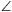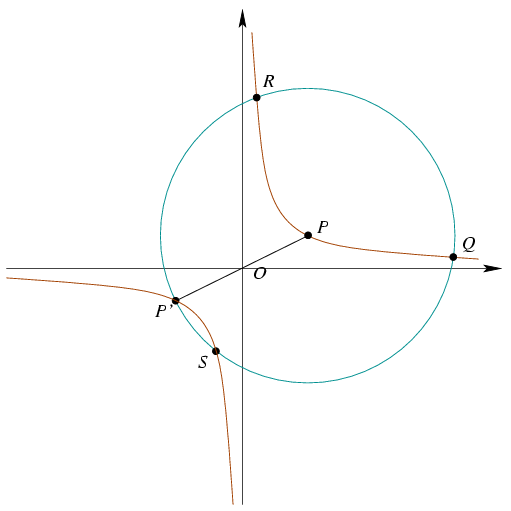Mathematical and Physical Journal
for High Schools
Issued by the MATFUND Foundation
 Already signed up? New to KöMaL?

#Problem A. 386. (December 2005)

A. 386. Consider one of the two arcs of a rectangular hyperbola centred at O, and choose an arbitrary point P on it. Let Q and R denote the intersections of the hyperbola and the circle of radius 2OP drawn about P. Prove thatQPR=120o.

(5 pont)

Deadline expired on January 16, 2006.

Solution. Place the hyperbola into the Cartesian co-ordinate system such that its equation is xy=1. Draw the other branch of the hyperbola as well. The circle and the second branch have another 2 intersections: S and P' which is the reflection of P to O.Let P=(a,b) and compute the intersections of the hyperbola and the circle. A point (x,y) lies on both curves if and only if

xy=1

and

(x-a)2+(y-b)2=4(a2+b2).

Thenx4-2ax3-3(a2+b2)x2-2bx+1=0. (1)

Let P'=(-a,-b), Q=(q1,q2), R=(r1,r2) and S=(s1,s2). The roots of the quartic equation (1) are exactly -a, q1, r1 and s1.

From Viéte's formulas, the sum of roots is 2a. Therefore

 q1+r1+s1=3a. (2)

Similarly,

 q2+r2+s2=3b. (3)

Equations (2) and (3) together yield that P is the centroid of triangle QRS. But P is the circumcenter as well. This implies that triangle QRS is equilateral, point P is its center anfQPR=120o.

### Statistics:

 14 students sent a solution. 5 points: Bogár 560 Péter, Erdélyi Márton, Estélyi István, Hujter Bálint, Jankó Zsuzsanna, Kisfaludi-Bak Sándor, Kónya 495 Gábor, Nagy 224 Csaba, Paulin Roland, Szakács Nóra, Tomon István. 4 points: Dücső Márton. 2 points: 1 student. 0 point: 1 student.

Problems in Mathematics of KöMaL, December 2005